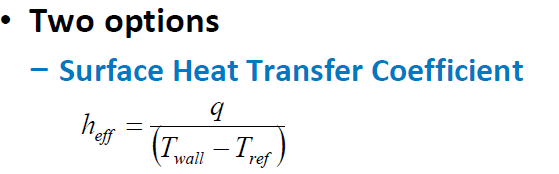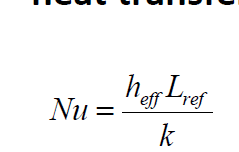# setting a reference temperature for htc and nusselt

Hi,

There are a couple of questions existent on the forum on this mater, but do not discuss temperature.
L03_ForcedConvection.pdf for Fluent Heat transfer pdfs does talk about it, but in that case, the bulk temperature itself is given as the reference temperature.

Could you please slightly elaborate, perhaps by giving a hypothetical example yourself, on how is the reference temperature to be given for nusselt calculations, lets say if I have a constant heat flux wall bc, instead of the T_wall bc specified in the above pdf.

As h_eff = q/ (T_wall - T_ref), and following the instructions of aforementioned pdf, I had set my reference temperature equal to bulk temperature at periodic bc, however this just gave a nusselt value (area-weighted average-on wall), twice of what I sould get from an emperical sieder-tate equation for a smooth pipe.

So the question is, because the pipe is smooth, and the reynolds turbulent, I should be able to get within 10% of an emperical correlation, but that is not the case, because the reference value setting for the htc, and hence the nusselt, needs an understanding.

• edited January 29

If you set a fixed flux the wall temperature will alter to pull the required amount of heat out of the solution. Nothing to do with the reference temperature.

• So, the pdf mentions the following two formulas" alt="" width="308" height="96"> and" alt="" width="160" height="102">, and later mentions" alt="" width="387" height="68">

So the point i am trying to make is, Nu will change, as per any T_ref value that I might enter.
So how can I know which value to enter?
Or what method can I perform (even manually) to come to this value.

And if the pdf is to be followed, setting the inlet temperature for periodic bc same as the reference temperature, does not seem to give me a correct Nusselt, or even nearby nusselt to an emperical relation. It is possible that, then I must find the emperical relation that is more close to the nusselt that I have obtained, but I wanted to know if there is a way for the other way around.

• edited January 30

Yes. You need to decide what value to use. Given it's a  made up number you can use any value you want and then define that.